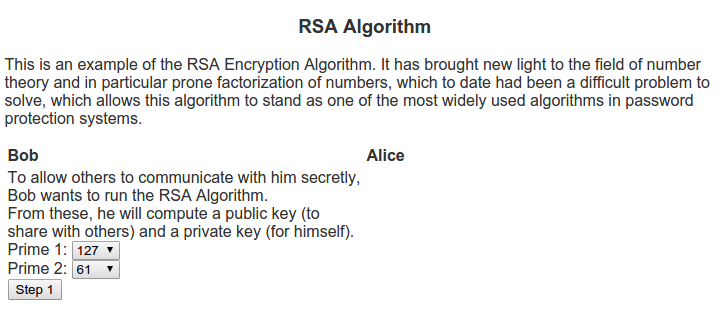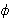# The RSA AlgorithmI can remember back when I was in school, still deciding whether I wanted to study pure or applied mathematics. One of the common questions I would receive from those in applied mathematical realms would sound like “What’s the point of doing mathematics with no real world applications?”. Generally my response to these questions was about the intrinsic beauty of mathematics, no different from an artist painting not for some desire to be a millionaire, but because of an burning desire to paint. Whether their paintings would one day be on the walls of a Smithsonian museum or sit on their mother’s refrigerator is generally outside of the thought process of the artist. So too, would I argue about the thought process of a pure mathematician.

When I was an undergrad and learned about the RSA algorithm (named for Ron Rivest, Adi Shamir, and Leonard Adleman who discovered the algorithm) it helped me explain this concept a lot better. The algorithm is based on prime numbers and the problem of finding the divisors of a given number. Many mathematicians throughout the ages have written papers on the beauty of prime numbers (see Euclid, Eratosthenes, Fermat, Goldbach, etc). For a large period in time one of the beautiful things about prime numbers was that they were so interesting in themselves. There were questions about how to check if a number is prime, question of patterns in primes, famous conjectures like the Goldbach conjecture and the twin prime conjecture, quick ways of finding prime numbers or numbers that are almost always prime, etc. In short, this was an active area of research that much of the applied world was not using. This all changed in 1977 when Rivest, Shamir and Adleman published the RSA algorithm.

The algorithm is in the area called public key cryptography. These algorithms differ from many of the previous cryptography algorithms, namely symmetric key cryptography. Whereas symmetric key cryptography depends uses the same device (key) to encode as to decode, public key cryptography creates two keys – one for encoding that is generally shared with others, and another for decoding which is kept private. These two keys in generally relate to a mathematical problem that is very difficult to solve.

In my example script for the RSA Algorithm, I show two people who want to communicate, Alice and Bob. Bob wants people to be able to send him messages securely so he decides to use the RSA algorithm. To do this, he first needs to think of two prime numbers, p1 and p2.
From these, he computes the following:
n = p1 * p2

Next, he computes Euler’sfunction on this n which can be calculated as(n) = (p1 – 1) * (p2 – 1)

Then Bob looks for a number e that is relatively prime to. This is what he will use as the encryption key.

One he has e, he can calculate d, which is the multiplicative inverse of e in(n).
This means that e * d = 1 (mod(n)).

The public key that will be used for encryption will be the pair (e, n). This is what he posts publicly via his web page for others to communicate with him securely. Bob also has a private key pair (d, n) that he will use to decrypt messages.

Alice sees Bob’s public key and would like to communicate with him. So she uses it to encode a message. The formula she uses to encrypt her message is c = me mod n, where c is the encrypted message. Once Alice encrypts her message, she sends it to Bob.

Bob receives this encoded message and uses the private key (d, n) to decode the message from Alice. The formula to decrypt is m = cd mod n.

For a more illustrative idea of how this algorithm works as well as examples, be sure to visit Script for the RSA Algorithm.

## 2 thoughts on “The RSA Algorithm”

1.torzsmokus says:

the script does not work properly for small primes :(

This site uses Akismet to reduce spam. Learn how your comment data is processed.